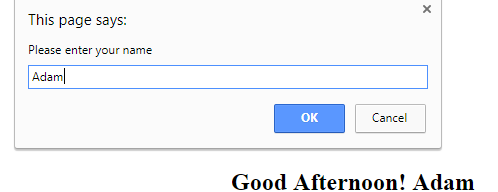## Decision Making in JavaScript

The assignment operator,=, is different from the equality operator (double equal),==. The following table shows the equality, relational and logical operators used in JavaScript:

### Equality and relational operators

```
Relational operator		Example in JavaScript		Meaning
==  					a==b    					a equal to b
===  					a===b    					a equal to b value and type (for example:
assume a = 9; a=== 9 is true but a==='9'
is false)
!=  					a!=b    					a is not equal to be
>					a>b					    	a is greater than be
<  					a<b					 	a is less than be
>=  					a>=b    					a is greater or equal to be b
<=  					a<=b    					a is less or equal to be

Logical operator			Meaning						Example in JavaScript
&&					   and						   (a < 7 && b > 5)
||					   or						   (a < 7 || b > 5)
!  					   not						   !(a == b)
```

### If statement

The next example shows how If statements are used to display greetings based on the current time of the day:
```	<!DOCTTYPE html>
<!--Example 1: If Statement and relational operators.html -->
<html>
<head>
<title> If Statement and relational operators </title>
<script >
<!--
var user_name = window.prompt("Please enter your name");
var c_time = new Date(); //current date and time
var c_hour = c_time.getHours(); //current hours (0-23)
//let's find out if it's morning from the 1st test
if (c_hour <12) document.write('<h2> Good Morning! </h2>'+ user_name + '</h2>');
//let's find out if it's afternoon/evening
if (c_hour >=12)
{
//let's see if the time is within afternoon and before evening
c_hour = c_hour-12;
if (c_hour < 6)
document.write('<h2> Good Afternoon! '+ user_name + '</h2>');
if (c_hour >= 6)
document.write('<h2> Good Evening! </h2>'+ user_name + '</h2>');
} //end if
// -->
</script>
</head>
<body></body>
</html>
```
See here a demo where time entered is in the afternoon:For more details, please contact me here.
Date of last modification: March 26, 2019.